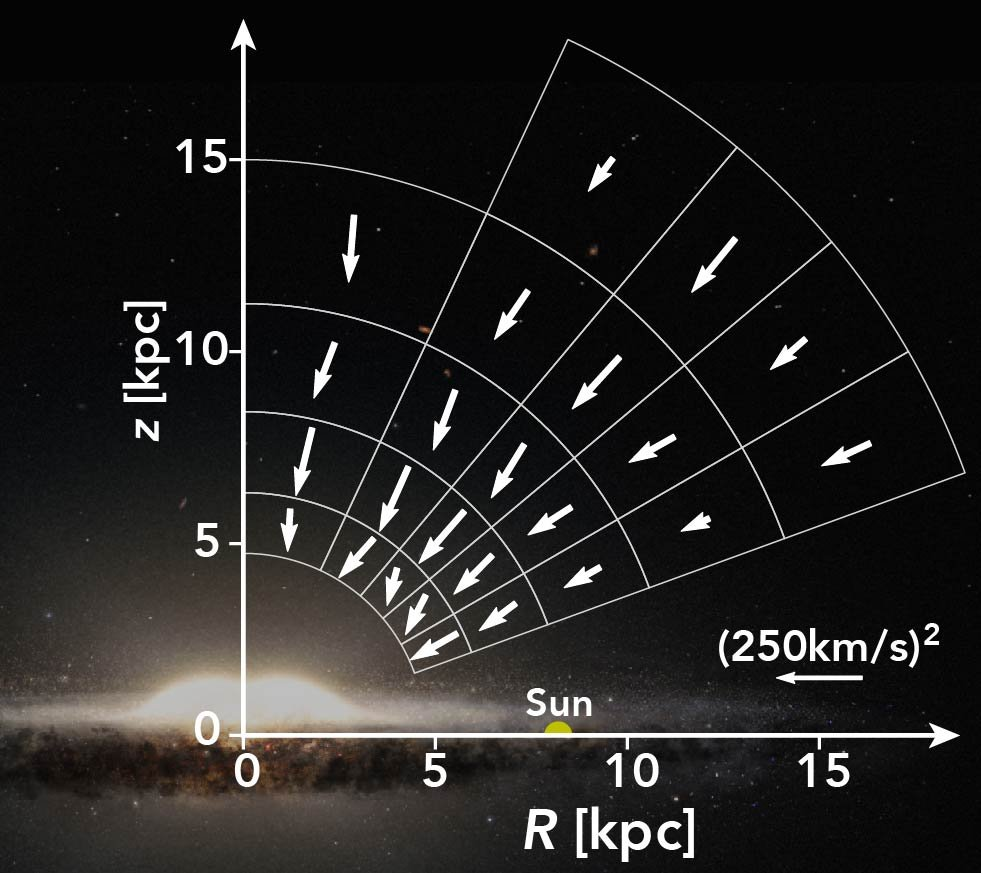# The Milky Way's gravitational force field measured from RR lyrae in gaia

## Wegg, C., Gerhard, O., Bieth, M., 2019, MNRAS, 485, 3296Arrows indicate the direction of the halo force field measured from RR Lyrae.

The Gaia satellite has measured proper motions (meaning the motion across the sky) for more than a billion stars across the Galaxy. However, unfortunately for most of these stars we do not have the distances we need to turn these proper motions into velocities. RR Lyrae are a type of variable star where we can estimate accurate distances. We have therefore used a sample of almost 16,000 RR Lyrae measured by Gaia to measure the dynamics of the stars which make up the Milky Way's stellar halo.

Because these stars have orbits of millions years the forces and accelerations are small, and the resultant changes in velocity are too small to be measured during our lifetime.  However we expect that the Milky Way is in equilibrium here, changing little with time. We can use this to understand what the gravitational forces in the Milky Way halo must be. For example if the velocities of the stars are high, then the gravitational forces must be strong, otherwise the Milky Way would not be held together and would fly apart in a relatively short timescale of less than a billion years. Therefore using this assumption of equilibrium, together with the velocities of our sample of RR Lyrae, we have measured the gravitational force field across the halo of our Galaxy for the first time (the force measurements are shown as the arrows in the figure).

The forces produced by the stars and gas we have see are insufficient to produce the entire force field, particularly in the outer regions there is a large amount of missing force: This missing force is the gravitational force produced by Dark Matter. By examining these forces we found that the dark matter which surrounds the Milky Way must be almost spherical in shape. This is surprising because most dark matter simulations predict the dark matter should be slightly flattened.